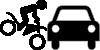# Motorcycle SafetyA person, of mass $m$, traveling on an indestructible motorcycle, of mass $M$, at a constant speed of $v$ collides elastically with an indestructible car that is not moving; what is the furthest this person will land from the motorcycle?

Details and Assumptions:

• To make the problem easier assume there is no resistance and disregard the possible height difference between the feet and the ground.
• Assume the motorcyclist launches at an angle $\theta = \pi/4$.
×